The OpenFOAM Foundation
polynomialFunction Class Reference

Polynomial function representation. More...

Inheritance diagram for polynomialFunction: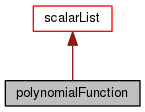[legend]
Collaboration diagram for polynomialFunction:[legend]

## Public Member Functions

TypeName ("polynomialFunction")
Runtime type information. More...

polynomialFunction (const label)
Construct a particular size, with all coefficients = 0.0. More...

polynomialFunction (const polynomialFunction &)
Copy constructor. More...

polynomialFunction (const UList< scalar > &coeffs)
Construct from a list of coefficients. More...

polynomialFunction (Istream &)
Construct from Istream. More...

virtual ~polynomialFunction ()
Destructor. More...

bool logActive () const
Return true if the log term is active. More...

scalar logCoeff () const
Return the log coefficient. More...

scalar value (const scalar x) const
Return polynomial value. More...

scalar integrate (const scalar x1, const scalar x2) const
Integrate between two values. More...

polynomialFunction integral (const scalar intConstant=0.0) const
Return integral coefficients. More...

polynomialFunction integralMinus1 (const scalar intConstant=0.0) const
Return integral coefficients when lowest order is -1. More...

polynomialFunctionoperator+= (const polynomialFunction &)

polynomialFunctionoperator-= (const polynomialFunction &)

polynomialFunctionoperator*= (const scalar)

polynomialFunctionoperator/= (const scalar)

## Friends

Ostreamoperator<< (Ostream &, const polynomialFunction &)
Ostream Operator. More...

## Detailed Description

Polynomial function representation.

poly = logCoeff*log(x) + sum(coeff_[i]*x^i)

where 0 <= i <= N

• integer powers, starting at zero
• value(x) to evaluate the poly for a given value
• integrate(x1, x2) between two scalar values
• integral() to return a new, integral coeff polynomial
• increases the size (order)
• integralMinus1() to return a new, integral coeff polynomial where the base poly starts at order -1
Foam::Polynomial for a templated implementation
Source files

Definition at line 72 of file polynomialFunction.H.

## Constructor & Destructor Documentation

 polynomialFunction ( const label order )
explicit

Construct a particular size, with all coefficients = 0.0.

Definition at line 84 of file polynomialFunction.C.

References UList< T >::empty(), Foam::exit(), Foam::FatalError, FatalErrorInFunction, and Foam::nl.

Here is the call graph for this function: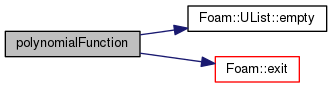polynomialFunction ( const polynomialFunction & poly )

Copy constructor.

Definition at line 99 of file polynomialFunction.C.

 polynomialFunction ( const UList< scalar > & coeffs )
explicit

Construct from a list of coefficients.

Definition at line 107 of file polynomialFunction.C.

References UList< T >::empty(), Foam::exit(), Foam::FatalError, FatalErrorInFunction, and Foam::nl.

Here is the call graph for this function:polynomialFunction ( Istream & is )

Construct from Istream.

Definition at line 122 of file polynomialFunction.C.

References UList< T >::empty(), Foam::exit(), Foam::FatalError, FatalErrorInFunction, and Foam::nl.

Here is the call graph for this function:~polynomialFunction ( )
virtual

Destructor.

Definition at line 139 of file polynomialFunction.C.

## Member Function Documentation

 TypeName ( "polynomialFunction" )

Runtime type information.

 bool logActive ( ) const

Return true if the log term is active.

Definition at line 145 of file polynomialFunction.C.

 Foam::scalar logCoeff ( ) const

Return the log coefficient.

Definition at line 151 of file polynomialFunction.C.

 Foam::scalar value ( const scalar x ) const

Return polynomial value.

Definition at line 157 of file polynomialFunction.C.

References polynomialFunction::integrate(), Foam::log(), List< T >::size(), and x.

Here is the call graph for this function: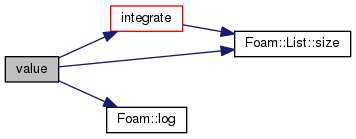Foam::scalar integrate ( const scalar x1, const scalar x2 ) const

Integrate between two values.

Definition at line 180 of file polynomialFunction.C.

References Foam::abort(), Foam::FatalError, FatalErrorInFunction, Foam::nl, and List< T >::size().

Referenced by polynomialFunction::value().

Here is the call graph for this function: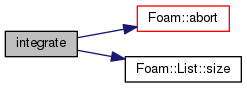Here is the caller graph for this function: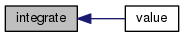Foam::polynomialFunction integral ( const scalar intConstant = `0.0` ) const

Return integral coefficients.

Argument becomes zeroth element (constant of integration)

Definition at line 211 of file polynomialFunction.C.

 Foam::polynomialFunction integralMinus1 ( const scalar intConstant = `0.0` ) const

Return integral coefficients when lowest order is -1.

Argument becomes zeroth element (constant of integration)

Definition at line 218 of file polynomialFunction.C.

 Foam::polynomialFunction & operator+= ( const polynomialFunction & poly )

Definition at line 227 of file polynomialFunction.C.

References forAll, List< T >::setSize(), and List< T >::size().

Here is the call graph for this function: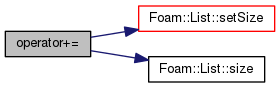Foam::polynomialFunction & operator-= ( const polynomialFunction & poly )

Definition at line 253 of file polynomialFunction.C.

References forAll, List< T >::setSize(), and List< T >::size().

Here is the call graph for this function: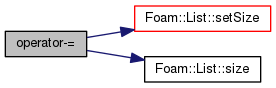Foam::polynomialFunction & operator*= ( const scalar s )

Definition at line 279 of file polynomialFunction.C.

References forAll, and s().

Here is the call graph for this function: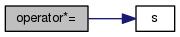Foam::polynomialFunction & operator/= ( const scalar s )

Definition at line 292 of file polynomialFunction.C.

References forAll, and s().

Here is the call graph for this function: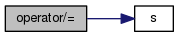## Friends And Related Function Documentation

 Ostream& operator<< ( Ostream & , const polynomialFunction & )
friend

Ostream Operator.

The documentation for this class was generated from the following files: